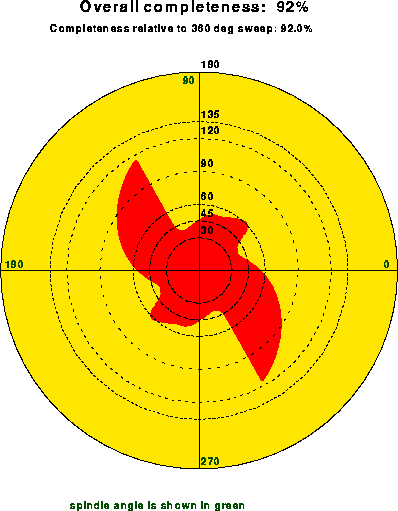Next: The X-File Up: Output Files Previous: The Log File

## The PostScript File

The minimal required total oscillation range as a function of the starting spindle angle will be displayed in a PostScript file. The name of this file is specified by the PSFIle command, the default is strategy.ps. The PostScript file contains a number of coloured figures, displaying the minimal required total oscillation range as a function of the starting spindle angle for the default and/or desired completeness values. The completeness is defined as the percentage of unique reflections that can be recorded. If the desired completeness is higher than the maximum completeness obtained by rotation of the crystal around the spindle axis for 360 degrees, the program will use the maximum completeness rather than the desired one.

Figureshows one page of the PostScript file.Figure: PostScript figure produced by STRATEGY

The figure is composed of a collection red vectors starting from the origin and representing the total minimal oscillation range (length of the vector) for a certain starting spindle angle (angle that the vector make with the horizontal axis). Seven black (dashed) circles are used to indicate the total oscillation range of 180, 135, 120, 90, 60, 45 and 30 degrees respectively. In green, four values of the starting spindle angle are given: 0, 90, 180 and 270 degrees.

The red patterns will be centrosymmetric if the ANOMalous option has not been used: Friedel's law can then be applied. The unique local minima of each figure will be listed in the log file produced by STRATEGY.

Home Page Crystal and Structural Chemistry